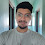# Taylor Swift - Delicate | Piano NotesTaylor Swift - Delicate | Piano Notes

This ain't for the best
C+AC+D+E+
My reputation's never been worse, so
D+D+D+D+D+E+ AAAAG
You must like me for me...
D+D+D+D+C+C+
We can't make
C+AC+D+E+
Any promises now, can we, babe?
D+D+D+D+D+E+ AAAAG
But you can make me a drink...
C+C+D+D+ D+D+C+C+

Dive bar on the east side, where you at?
D+D+ C+D+D+D+, C+D+D+
Phone lights up my nightstand in the black
D+D+ C+D+D+D+, C+D+D+
Come here, you can meet me in the back
D+D+ C+D+D+D+, C+D+D+
Dark jeans and your Nikes, look at you
D+D+ C+D+D+D+, C+D+D+
Oh damn, never seen that color blue
D+D+ C+D+D+D+, C+D+D+
Just think of the fun things we could do
D+D+ C+D+D+D+, C+D+D+

'Cause I like you
This ain't for the best
C+AC+D+E+
My reputation's never been worse, so
D+D+D+D+D+E+ AAAAG
You must like me for me...
D+D+D+D+C+C+

Yeah, I want you
We can't make
C+AC+D+E+
Any promises now, can we, babe?
D+D+D+D+D+E+ AAAAG
But you can make me a drink...
C+C+D+D+ D+D+C+C+

Is it cool that I said all that?
C+D+D+ D+D+E+C+A
Is it chill that you're in my head?
C+D+D+ D+D+E+C+A
'Cause I know that it's delicate (delicate)
C+D+D+ D+D+E+C+A (E+C+A)
Is it cool that I said all that
C+D+D+ D+D+E+C+A
Is it too soon to do this yet?
C+D+D+ D+D+E+C+A
'Cause I know that it's delicate
C+D+D+ D+D+E+C+A

Isn't it? Isn't it? Isn't it?
AC+C+ AC+C+ AC+C+
Isn't it?
AC+C+
[2 TIMES]
Delicate
E+C+A

Third floor on the west side, me and you
D+D+ C+D+D+D+, C+D+D+
Handsome, you're a mansion with a view
D+D+ C+D+D+D+, C+D+D+
Do the girls back home touch you like I do?
C+D+D+D+D+D+D+, C+D+D+
Long night, with your hands up in my hair
D+D+ C+D+D+D+, C+D+D+
Echoes of your footsteps on the stairs
D+D+ C+D+D+D+, C+D+D+
Stay here, honey, I don't wanna share
C+D+D+D+D+D+D+, C+D+D+

'Cause I like you
This ain't for the best
C+AC+D+E+
My reputation's never been worse, so
D+D+D+D+D+E+ AAAAG
You must like me for me...
D+D+D+D+C+C+

Yeah, I want you
We can't make
C+AC+D+E+
Any promises now, can we, babe?
D+D+D+D+D+E+ AAAAG
But you can make me a drink...
C+C+D+D+ D+D+C+C+

Is it cool that I said all that?
C+D+D+ D+D+E+C+A
Is it chill that you're in my head?
C+D+D+ D+D+E+C+A
'Cause I know that it's delicate (delicate)
C+D+D+ D+D+E+C+A (E+C+A)
Is it cool that I said all that
C+D+D+ D+D+E+C+A
Is it too soon to do this yet?
C+D+D+ D+D+E+C+A
'Cause I know that it's delicate
C+D+D+ D+D+E+C+A

Isn't it? Isn't it? Isn't it?
AC+C+ AC+C+ AC+C+
Isn't it?
AC+C+
[2 TIMES]
Delicate
E+C+A

Sometimes I wonder when you sleep
G+G+G+G+G+ G+E+G+A+
Are you ever dreaming of me?
G+G+G+G+ G+G+E+G+A+
Sometimes when I look into your eyes
G+G+G+G+G+ G+G+E+A+
I pretend you're mine, all the damn time
G+G+G+G+G+, G+G+G+A+

('Cause I like you
Is it cool that I said all that?
C+D+D+ D+D+E+C+A
Is it chill that you're in my head?
C+D+D+ D+D+E+C+A
'Cause I know that it's delicate (delicate)
C+D+D+ D+D+E+C+A (E+C+A)
(Yeah, I want you
Is it cool that I said all that
C+D+D+ D+D+E+C+A
Is it too soon to do this yet?
C+D+D+ D+D+E+C+A
'Cause I know that it's delicate (delicate)
C+D+D+ D+D+E+C+A (E+C+A)

'Cause I like you
Is it cool that I said all that?
C+D+D+ D+D+E+C+A
(Isn't it?
AC+C+)
Is it chill that you're in my head?
C+D+D+ D+D+E+C+A
(Isn't it? Isn't it?
AC+C+ AC+C+)
'Cause I know that it's delicate (Delicate)
C+D+D+ D+D+E+C+A (E+C+A)
(Isn't it?
AC+C+)

(Yeah, I want you
Is it cool that I said all that
C+D+D+ D+D+E+C+A
(Isn't it?
AC+C+)
Is it too soon to do this yet?
C+D+D+ D+D+E+C+A
(Isn't it? Isn't it?
AC+C+ AC+C+)
'Cause I know that it's delicate
C+D+D+ D+D+E+C+A
(Isn't it?
AC+C+)
Delicate
E+C+A

1.